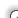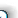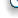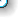It is currently Sat Sep 30, 2023 7:58 am

 All times are UTC - 5 hours

 Page 1 of 2 [ 23 posts ] Go to page 1, 2  NextAuthor Message
 TOC Moderator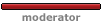Joined: Wed May 18, 2005 5:42 pm
Posts: 1297
Images: 2
Location: Ames, IA
 Brando and all, I have noticed alot of electrical problems lately, and some unintentional misinformation on electrical repairs and theory. So I thought I would post some stuff on electricity for us all to use. Maybe if you like this, it could become a "sticky" Ill make seperate posts on this thread so it is all located in one place. To start with, all matter is composed of atoms, which are composed of some basic particles of electric charges. The smallest and lightest are called electrons and are referred to as negative charges. The positive particle is known as the proton. As far as is known, all matter is made up of these particles. Some of the characteristics are: like charges repel each other and unlike charges attract. This is similar to magnets, where like poles repel each other and unlike poles attract. A small experiment you could perform to see this would be to take a metal rod and attach 2 leaves of tin foil to one end and hang it in a glass jar. If you would take a glass or plastic rod and rub it with some fur to charge it up, then touch it to the metal rod, the 2 foil leaves will spread apart. This is due to the fact that that the static charge built up on the rod charges both leaves of the foil and they will physically move apart. Atoms are composed of a nucleus containing protons that are 1840 times heavier than electrons. Around the nucleus are an equal number of electrons flying around the nucleus like planets orbit the sun in our solar system. How these electrons are arranged determines the stability of the atom. The electrons are in different orbits, but the electrons in the outermost orbit are the ones that we will be observing. For example the hydrogen atom has 1 proton and 1 electron spinning around it making it the lightest atom. Typically the first ring is stable with 2 electrons. The pull on the electron by the proton is offset by the centrifugal force of the electron flying around the proton keeping it in orbit. Since it has one electron and 1 proton, its atomic weight is 1 and the elements go to uranium having an atomic weight of 92 with 92 protons and 92 electrons. Typically the electrons in the outer ring needs to number eight for stability. Lets look at a couple of atoms that are common in the electrical world. The carbon atom has 6 protons in its nucleus, 2 electrons in the first ring and 4 in the second. The copper atom has 29 protons and 2 electrons in the first ring, 8 in the second, 18 in the third and 1 in the forth. Atoms that do not have 8 electrons in the outside orbit are not stable electrically. The farther from 8, the less stable. In the above examples the outer orbit of copper having 1 electron is less stable than the carbon atom with 4 electrons in the outer orbit. This is a very complex subject and I have picked a couple of atoms and just tried to illustrate what basically happens. If you desire any more information on this subject, get a good Physics book and it will explain it a lot better than I can in this small space. Keep in mind that the sketch of atoms, below, appears like the orbits are all on the same flat plane. In reality electrons are like tennis balls and their orbits, which look like wire grids, make up the "shell" of the atom. As another example, think of our solar system, and picture the sun as the nucleus of the atom and the planets as the electrons.Now we will look at why these things are significant. If you take a copper wire made of "a gazillion" atoms and put a positive charge at one end this charge will attract one of the unstable electrons from the outer orbit of one of the atoms. This will leave this atom with one more protonthan electron, so it will be unbalanced by 1 positive charge. T his atom will attract an electron from the outer ring of an adjacent atom. The missing electron gets replaced by one from the outer orbit of an adjacent atom. This activity continues from atom to atom creating an electric current. The more positive the charge at the end of the wire the more electrons are moved. The carbon atom with 4 electrons in its outer ring or valence is more stable than copper with only 1 electron. If the same positive charge is applied to a carbon rod, since it is more stable fewer electrons will be attracted and the flow of electrons will be significantly less. Therefore copper is a much better "CONDUCTOR" of electricity than carbon. Also the thicker the material the more atoms there to give and receiver electrons, so the greater capacity for electron flow. An atom that has 8 electrons in its outer ring or valence is stable and the electrons will not leave the orbit in response to a positive charge. This material is called an "INSULATOR". (These materials are also called DIELECTRICS.) When an electric current is flowing in a conductor another curious thing occurs. The flow of electrons creates a magnetic field around the conductor. Conversely another unique thing happens. If you move a magnetic field across a conductor it causes the electrons to flow the same as applying a charge at the end of the conductor does. What is happening is we are converting mechanical energy to electrical energy. As we get into this deeper we will see how these factors are adapted to motors, generators, solenoids, transformers, ignition coils, voltage regulators etc. THERE IS ONE VERY CONFUSING ITEM: ELECTRON FLOW IS FROM NEGATIVE TO POSITIVE. IN GENERAL PRACTICE CURRENT FLOW IS SAID TO BE FROM POSITIVE TO NEGATIVE. In normal discussion people usually think of current flowing from positive to negative, and as we go forward, we will probably use that terminology. I think it is important that we have an understanding about atomically what an electric current is. There is one other law of physics we must remember and that is: "Energy can neither be created or destroyed, but may be transformed." So as we journey forward, remember that electron flow may be transformed to mechanical energy, heat, etc. and these items may be transformed into electron flow. Last edited by andrewk on Thu Oct 13, 2005 12:54 am, edited 2 times in total. _________________Andrew TOC Moderator Mark Twain wrote:A man's character may be learned from the adjectives which he habitually uses in conversation.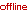Post subject: Part deux
 TOC ModeratorJoined: Wed May 18, 2005 5:42 pm
Posts: 1297
Images: 2
Location: Ames, IA
 We have discussed very briefly what electric current is and how it works. Now we will move to adapting the theory to more practical applications. We will discuss conductors, resistors and insulators, and how electrical properties are measured. We will limit the scope in this section to DC applications only. In dealing with electricity, there some terms that you must know and the relationship between them. If you understand these basics you will be able to analyze what is happening in a circuit when you are trying to troubleshoot it. E = Electromotive force, also called Voltage, measured in Volts. I = The flow rate of electricity, also called Current, measured in Amperes. R = Resistance, the opposition to current flow, measured in Ohms. P = Power, the quantity of electric energy, measured in Watts. To illustrate the relationships, it is often easier to visualize if you compare it to water. For example, if you had a dam and at the bottom you drilled a hole through it and put a pipe in it. If the pipe was capped you would have pressure on the pipe relative to the height of the column of water above it. This pressure in water is measured in PSI (pounds per square inch). Because the pipe is capped and there is no flow this is "potential energy". This corresponds to electromotive force (EMF) or Voltage, with the symbol V. Next, let's uncap the pipe. We now have water flowing through it. This flow is measured in Gallons Per Minute (GPM). This corresponds to current or the flow of electrons measured in Amperes, with the symbol I. Now, let's look at the size of the pipe. The larger the pipe the less resistance to the flow, so the more Gallons Per Hour (GPH). The longer the pipe, the more friction on the walls and the less water flows through it. So, both the size and the length of the pipe affect the flow rate (GPH). This corresponds to Resistance, measured in Ohms. The symbol for Ohms is the capital Omega symbol, W. The longer the wire or the smaller its size, the higher its resistance to the flow of current. If we want greater a volume of water delivered in a given time, we can do two things. One, we could raise the dam so the water column would be higher and the pressure would increase. Increased pressure would push more water through the pipe. The second option would to use a larger diameter pipe at the original level and by reducing the resistance we could increase the flow, or GPH. Electricity works in the same way. To increase the electrical current (measured in amperes), we can increase the electromotive force (measured in volts) or decrease the resistance (measured in ohms). Next we need to look at Power. If we hook the pipe to a hydraulic motor connected to a load, the amount of work that can be extracted from the motor (horsepower) is relative to the pressure PSI on the water and the rate of flow GPH. This corresponds to electric power, measured in Watts (W) or in the case of our homes, Kilowatt Hours, or KWH. Kilowatt hours equal Watts X Hours X 1000. Horsepower is often expressed in Kilowatts, particularly in Europe. One often sees engines rated as so many kilowatts [KW]. One horsepower is equal to 746 watts of electricity. A 3KW engine would be about the same as 4HP. A corresponding example would be a tractor you need to start. You have a 12v battery and you use a small wire and hook it to the starter. Because the small wire has a lot of resistance, most of the energy will be lost in the wire and the engine won’t crank because not enough energy is getting to the starter. The wire will possibly burn because most of the energy is lost in the wire. Now if we take a large cable and hook it up, it will have a very low resistance and most of the energy will get to the starter and crank the engine. This works just like the small pipe and the large pipe, and the same with the length of the pipe. See, it is not that mysterious! It is just that we can see the water and we can’t see the building blocks of atoms moving, so it is easier to comprehend examples using water. _________________Andrew TOC Moderator Mark Twain wrote:A man's character may be learned from the adjectives which he habitually uses in conversation.Post subject:
 TOC ModeratorJoined: Wed May 18, 2005 5:42 pm
Posts: 1297
Images: 2
Location: Ames, IA
 To understand electricity, one needs to be familiar with the common measurements and what they are. Volts: The unit of measure of electromotive force, E in Ohms Law 1 Microvolt = .000001 volt = 1 uv. = one 1/millionth of a volt 1 Millivolt = .001 volt = 1 mv. = one 1/thousandth of a volt 1 Volt =1 volt = 1 v. 1 Kilovolt = 1000 volts = 1 kv. Amperes: The unit of measure of electric current, I in Ohms Law 1 Ampere = 1 amp. = 1a. 1 Milliampere = .001 amp. = 1 ma. = one 1/thousandth of an ampere 1 Microampere= .000001 amp. = 1 ua. = one 1/millionth of an ampere Ohms: The unit measure of resistance, R in Ohms Law 1 Ohm = 1 ohm [omega symbol, not on keyboard] 1 Kilohm = 1 k ohms = 1000 ohms 1 Megohm = 1 meg. or 1 m. = 1 Million Ohms Watt: The unit of measure of electrical Power 1 Microwatt = .000001 watt = 1 uw. = one 1/millionth of a watt 1 Milliwatt = .001 watt = 1 mw = one 1/thousandth of a watt 1 Watt = 1 watt =1 w. 1 Kilowatt = 1000 watts =1 kw = 1 thousand watts 1 Megawatt = 1000000 watts = 1 megw = 1 million watts Horsepower: 746 Watts = the amount of energy in 1 horsepower There are only 2 formulas that you need to know and understand, in order to solve most common electrical problems on small power equipment. The first is Ohms Law: E = IR. This says that Voltage = Current X Resistance. Conversely, you can Find I with I = E/R and R = E/I. The second is the Power formula: P = EI. This says that Power in Watts = Voltage X Current (Note: current is often called amperage). Conversely, E = P/I or I = P/E and P = E2/R and P = I2R. Now, let's apply these formulas to a couple of tractor examples to see how they work. Example 1: Your tractor has two 12 volt headlights rated at 36 watts each, connected in parallel. Your tractor has a 6 amp charging system. You would like to add two more lights, can you do this with the charging system you have? There are two ways to solve this. 1st Method, using the Power formula: P, or Power = 12volt system, so E [12 Volts] X I [Current 6 amps.] = 72 Watts. This is the capability of the charging system. Two lamps in parallel with 36 watts each = 72 watts. No extra power is available for more lights. Connecting more light would result in the battery not staying charged and likely "smoke" the rectifier or stator in the charging system. 2nd Method, using Ohm's Law: Your lights are rated at 36 Watts. Current available, I = P [36W] /E [12V], so Current, or I = 3 amps each. Two lamps, at 3 amps each, equals 6 amps, the total capacity of the charging system. Example 2: Your mower has an electric clutch with 4 ohms resistance and the two lights above. You are looking at a replacement engine. What size alternator does it need? To solve this we need to use Ohms Law to find how much current the clutch draws. You have a 12 volt system, so: I, Current = E [12 Volts] / R [4 ohms] = 3 Amps You would need a charging system that would provide current to charge the battery after starting, plus 3 Amps for the clutch, plus 6 Amps for the lights or more than a 9 Amp charging system. In this case an engine with a 6 amp alternator would not be a deal even if it was cheap, as it would need to be converted to a different charging system. Next I will go to some basic circuits, and explain how resistance, voltage and current interact in a circuit. Once you understand these principles, how you would use a voltmeter to locate troubleshoot wiring problems in you tractor, or actually any electric circuit. First we need to know some common symbols and what they are. Then later we can use them to draw a diagram and analyze how it works.These symbols are used to create schematic diagrams, these show electrically how a circuit works. Some industrial and other diagrams use some variation of these symbols. In the next article  we will look at series and parallel circuits and how they work, using some of these symbols. _________________Andrew TOC Moderator Mark Twain wrote:A man's character may be learned from the adjectives which he habitually uses in conversation.Post subject:
 TOC ModeratorJoined: Wed May 18, 2005 5:42 pm
Posts: 1297
Images: 2
Location: Ames, IA
 n this section we will look at some simple DC series and parallel circuits. The purpose will be to observe the relationships occurring between Voltage, Current, Resistance and power. We will explore how changing the Voltage affects the Current and the fact that Power is a square law function relative to Voltage and Current. In all of these examples we will assume that there is NO resistance in the wires, ammeter or the battery [Not possible in the real world] . Figure 1, below, demonstrates a simple complete circuit with one resistor. It could be viewed as a Series circuit because it is in series with the ammeter, and could also be considered a Parallel circuit because the load resistor is connected directly across the battery.Example1 1 P = EI = 10W E = IR if V = ??, I = 1 Amp, R = 10 W A:10V Example2 2 P = EI = 40W I = E/R if V = 20V, I = ?? Amps, R = 10 W A:2 Amps Example3 3 P = EI = 576W R = E/I if V = 12V, I = 48 Amps, R = ?? W A:25 W In this circuit the current flows from the Battery through the Ammeter, R1 and back to the Battery. Note the current is equal in all parts of this circuit. In the first example, if the voltage was unknown, we could find it using Ohms Law E = IR. [E] ?? = 1a [I] X 10 ohms [R] = 10 volts The Power [P] is found using the Power formula P=EI P = [E]10V X [I] 1a. = 10 Watts In the 2nd example we will change the Battery Voltage to 20v and leave the resistor 10 ohms. Now to find the current this circuit will draw we will use Ohms Law again. I = E/R [I] ?? = [E] 20v / [R] 10 ohms = 2 amps Now if we calculate the Power P=EI we see that: [P] ?? = [E] 20v X [I] 2 a. = 40 watts Note that when the Source Voltage is doubled and the Resistance remains constant, The Power increases by 4. This is a "Square Law" function. If the Voltage had been increased by 3 times the Power would have increased by 9 times. In the 3rd example we have a 12 Volt Battery and the ammeter is indicating 48 amps of current flowing. What is the value of R1? [R] ?? = [E] 12 v /48a [I] = .25 ohm The Power would then be: [P] ?? = [E] 12v X [I] 48a = 576 watts The purpose of this exercise was to illustrate the effects of changing Voltage, Current and Resistance in a circuit and their relationship to each other and to observe how these changes affect the total Power. Figure 2, below, illustrates two resistors connected in series. Note that in this circuit the Current will be the same in all parts of the circuit.In this circuit the current path is from the battery through the ammeter through R1 then R2 then back to the battery. The value of the two resistors ADD together when they are in SERIES: R1 + R2 = 5 + 15 = 20 ohms With 20 volts applied, E = IR = 20 v = 1a [I] X 20 ohms [R]. Note: The voltage drop across R1 would be 5 V, as shown by: [E] 5v = [I] 1a X [R] 5 ohms The same would be true for R2. NOTE: The voltage drop in a SERIES circuit is always proportional to the resistance. If one was to assume R2 was a light and that R1 was a piece of wire that had 5 ohms resistance, it is obvious that ¼ of the power would be lost getting to the light. This is how one adapts or utilizes these concepts in practical applications. If you understand what happens in a circuit like this, then when you take a voltmeter and start measuring you can understand what it is telling you and locate the problems. Figure 3, below, illustrates a circuit with 2 resistors in parallel, and what happens.In this circuit we will not go through all of the E = IR calculations. Note that Both Resistors have 20 volts across them. If we take the Current flowing through R1, which is 1amp, and the Current flowing through R2 and add them together we get 1a + 4a = 5a, or the total circuit current is 5a. If we look at E = IR, then: 20v @ 5a = 4 ohms. Using P = EI the total power provided by the battery is: 20v X 5a =100 watts Of these 5 watts are dissipated as heat in R2 and 20 are dissipated in R1. Note the parallel resistance formula in line 4, calculates to 4 ohms which agrees with the power calculations previously done. If more than 2 resistors are connected in parallel the formula would be the one shown in the bottom line. Note: IN THIS CIRCUIT WITH 2 RESISTORS IN PARALLEL THE CURRENT THROUGH THE RESISTORS IS INVERSELY PROPORTIONAL TO THE RESISTANCE OF THE RESISTORS. Note also that when we insert Ohms Law [E = IR, and I = E/R] into the Power Formula: P = EI, then P = [IR]I, therefore P = [I]2 R. If we insert P = E[E/R], we get the formula P = [E] 2/R. You can see these calculations used in line 2 and 3 above. This circuit should demonstrate how current flow is inverse to resistance values in parallel circuits and that the current flow is not the same in all parts of the circuit. Further Note: If a resistor were to replace the ammeter, you would have a series parallel circuit and the 2 parallel resistors R1 and R2 would look like a 4 ohm resistor to the series resistor and the voltage drop across the two would be proportional to added resistor and the 4 ohms for this pair. _________________Andrew TOC Moderator Mark Twain wrote:A man's character may be learned from the adjectives which he habitually uses in conversation.Post subject:
 TOC ModeratorJoined: Wed May 18, 2005 5:42 pm
Posts: 1297
Images: 2
Location: Ames, IA
 This article will deal with the relationship between magnetism and electric current. We will explore how mechanical energy can be converted to electrical energy, how electrical energy can be converted to mechanical energy and the many applications where this is used. The Earth has a giant magnetic field with one of the poles located about 15 degrees from its true axis of rotation at the North and the South Pole. This is referred to as Magnetic North Pole. If we take a needle which is hard steel and stroke it repetitively across the pole of a magnet it will become magnetized. Now if we take a piece of string, and tie a knot around the needle so it hangs horizontally and the string is long and soft enough to let it turn freely enough, one end will point North. We could do the experiment with a small disc of cork in a glass of water and lay the needle on it. Using either method, we have created a "compass." The end that seeks the north would be called the North pole. The North seeking pole is always referred to as the North pole of a magnet. Magnetism got its name from the properties of the iron oxide material magnetite. Magnetic properties that refer specifically to the magnetic properties of iron are referred to as ferromagnetic. Materials made from iron, nickel and cobalt are particularly able to concentrate their magnetic fields at opposite ends. Soft iron does not retain its magnetic properties nearly as long as harder steel and various alloys. The ability to concentrate magnetic lines of force is called permeability. The measure of relative permeability is always stated as one magnet to another. When we investigate electromagnets, we will explore this a little further.Figure 1-1. This figure shows a couple of typical magnets. Note that the lines of force are concentrated between the poles in the horseshoe magnet. They are dispersed in the air gap, or the space between the poles. In Figure 1-2 we see a bar magnet. The lines of force are concentrated in the pole pieces and loop around to the opposite pole piece. If you took a nail and placed it close to either end there would be a strong pull, if you placed it in the center there would be almost no pull at all because the lines of force are not concentrated there. One can easily to see the lines of force by taking a sheet of paper and sprinkling iron filings on it; then put a magnet under it. You will be able to see it in 3-dimensions. If you need a source of iron filings, find someone that grinds saw chains and take a magnet you can get all you need off the chain grinder. Figure 1-3. We have 2 bar magnets placed with opposite polarities together. Placed this way the magnets are attracted to together because opposite poles attract. Opposite poles attract. If the magnets are stored this way they will retain their magnetism for a much longer period of time. If you put iron filings around them you would see very little external field effect, as the field would be concentrated in the two magnets. Figure 1-4. Two bar magnets are placed together with like poles together. The magnets will repel each other. Like poles repel. If these magnets were stored in this manner and were forced close together, they would de-magnetize each other.Figure 2a. This sketch represents a wire with current flowing through it. When current flows through a wire magnetic fields are set up around the conductor. These lines of force are circular around the conductor. To observe this, take a wire that is going to a load, for example, a wire from a 12v battery to a headlamp unit. Poke a hole in a sheet of paper and run the wire through it vertically. While current is flowing to the light, pour some iron filings [thin layer] around the wire. Lightly tap the paper and observe the pattern of the Iron filings. You will be able to see the effects of the magnetic field. Looking at Figure 2d observe the Left Hand Rule. If you moved the hand up to 2a, the thumb would point to the positive end of the wire also the North Magnetic pole. The fingers point in the direction of rotation of the magnetic lines of force that surround the conductor. Figure 2b. We have taken the wire and wound it in a coil with adjacent turns. The individual force fields around each turn combine with the field of the adjacent wire and add to the field. The result is a field for the coil that comes out of the center and loops around like the bar magnet to the other end. This type of coil doesn’t concentrate the lines of force very well. In Figure 2c we will add an iron core. If the core is free to move it will center itself in the coil and will concentrate the force field in each end, the behavior will be like the bar magnet. The difference is that when the voltage applied to X and Y is removed, the magnetic field will collapse and all that will remain is the residual magnetism in the core. If the core is soft iron very little magnetism will remain. This is an electromagnet and its magnetic power is relative to the amount of power dissipated in the coil [Back to Ohms Law and the Power Formula] plus some qualities and the quantity of the core material. In Figure 2a when the voltage is applied to the coil, [let's assume that the resistance of the coil is such that with this source 1 amp would flow] the current doesn’t reach the 1 amp instantly like it does in a resistive circuit. The first thing that has to happen is for the magnetic field to develop, so the full voltage is applied to the ends of the coil and as the core of the coil becomes magnetically saturated the current is at the level of the resistance of the wire. This is called Inductance and is measured in Henrys in quantities ranging as low as picohenrys or 1/1000 of 1/1,000,000th of a henry. Inductance tends to resist current changes. The relationship between voltage, current, time and resistance is called inductive reactance. This is a very involved subject, and I will not get into it any deeper right now. One other effect that MUST be mentioned now is BEM, Back Electromotive Force. When we remove the voltage source from the coil, all of the magnetic lines of force instantly collapse and as they cut the wires they induce a voltage spike that is many times higher than the voltage that was applied to the coil. Later when we get to ignition systems we will explore how this works and how we use it to generate very high voltages for ignition. When a conductor is moved through magnetic lines of force, and cut the lines of force, or the lines of force cross the conductor and are cut by it in a collapsing field, the voltage produced is said to be induced. And this is called induction.Figure 3a illustrates a horseshoe magnet with a wire in the force field. If the wire is moved up and down so it cuts the magnetic field will cause electrons to move from one atom to another. As long as the wire is not moving no activity will transpire. When the wire is moved, mechanical energy will be converted to electrical energy and a voltage potential will appear at A and B with one being positive and the other being negative. Figure 3b. The situation is the same as 3a except we have added 3 more wires in the field by winding a coil. Since we have 3 wires cutting the lines of force, and they are in series the voltage developed at A and B will be 3 times what it would be with the 1 wire in 3a. This is the principle that allows electricity to be generated from mechanical energy. The faster the conductor is moved, cutting the magnetic lines of force the more voltage is generated. This process is called induction. Generators, alternators, ignition systems, transformers all work on induction, or induced currents. Later when we get deeper into generator and alternator principles we will see how this action is expanded into multi-polar devices and how alternating current works. The next article will address how these principles are used in motors, solenoids, generators and other items. _________________Andrew TOC Moderator Mark Twain wrote:A man's character may be learned from the adjectives which he habitually uses in conversation.Post subject:
 TOC ModeratorJoined: Wed May 18, 2005 5:42 pm
Posts: 1297
Images: 2
Location: Ames, IA
 This is alot of material. My old boss wrote all this stuff down, with a bit of my help, and he taught it all to me. his site is www.edensltd.com if you want to see this stuff in its original form. Hopefully this helps out! Andrew _________________Andrew TOC Moderator Mark Twain wrote:A man's character may be learned from the adjectives which he habitually uses in conversation.Post subject:
 TOC Member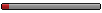Joined: Wed Oct 12, 2005 12:40 am
Posts: 37
Location: moorseville
 wow this stuff looks all too familiar. i dont know if i said it or not but i am currently attending a school called Nascar Technical Institute. i just got done with my corse on electronic fundamentals and have now started on electronic technology. this stuff is very accurate to what we are using great job on it man. -Intimidator68Post subject:
 TOC Member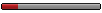Joined: Sun Jun 20, 2004 1:39 pm
Posts: 67
Location: San Antonio, TX
 Good stuff! Intimidator, where's the school at? Sounds like a cool deal. C.J.Post subject:
 TOC MemberJoined: Wed Oct 12, 2005 12:40 am
Posts: 37
Location: moorseville
 The school is in Moorseville N.C. It is about 20 miles North of Charlotte and about 20-30 miles southeast of Hickory. It is a great school and I have learned a whole lot more in the short time ive been there then i ever did in my home town -Intimidator68TOC Member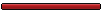Joined: Sun Feb 15, 2004 3:25 am
Posts: 3196
Images: 10
Location: Southern California
 Hi andrewk, hello everyone, Quote: ........ THERE IS ONE VERY CONFUSING ITEM: ELECTRON FLOW IS FROM NEGATIVE TO POSITIVE. IN GENERAL PRACTICE CURRENT FLOW IS SAID TO BE FROM POSITIVE TO NEGATIVE ......... Current flow in our Oldsmobiles, is from -ground to +battery? If you reverse the coil wires, you make the spark jump from ground to the center electrode on the plug, and the engine doesn't run very well. I believe the change from positive to negative ground, in automobiles, was to make the flow go to ground for a more efficient electrical system. Does that mean lightning goes from the earth (ground) to the clouds? Looks like good reason for the confusion.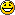Regards, Norm _________________Harry S. Truman wrote:When you have an efficient government, you have a dictatorship.Post subject:
 TOC MemberJoined: Sun Jun 20, 2004 1:39 pm
Posts: 67
Location: San Antonio, TX
 Norm and all, For the whole negative/positive thing, this is from one of my old texts: "Although the negative charged electrons move through the wire toward the positive (+) terminal of the source of electricity, the current is indicated as going from positive to negative. This is an unfortunate and confusing convention. Ben Franklin originally named charges positive (+) and negative (-) when he was studying static electricity. Later, when scientists were experimenting with electrical currents, they said that electricity travels from (+) to (-), and that became the convention. This was before electrons were discovered. In reality, the negative charged electrons move toward the positive, which is the opposite direction that people show current moving. It is confusing, but once a convention is made, it is difficult to correct it." For a good explaination of how that relates to spark plugs, look on page 3 here: http://www.mightyautoparts.com/pdf/articles/tt105.pdf I just try not to fill my head with too much and I'm ok.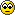C.J.TOC AdminJoined: Fri Jun 06, 2003 3:58 pm
Posts: 3166
Images: 29
Location: Michigan, USA
 88 Coupe wrote:Does that mean lightning goes from the earth (ground) to the clouds? yes And that IS a LOT of info, looks like last years physics class...Definately a sticky. TOC Admin Brando _________________1999 Oldsmobile Intrigue GLS1987 Oldsmobile Delta 88 RoyalePost subject:
 TOC ModeratorJoined: Wed May 18, 2005 5:42 pm
Posts: 1297
Images: 2
Location: Ames, IA
 Norm, on older cars and most farm tractors, current flow goes neg to pos. They use a positive ground system. When they changed from generators to alternators, the electrical system changed to negitive ground, IIRC. And yes, lightning goes from the earth to the clouds... speed of light is pretty damn fast to the human eye..._________________Andrew TOC Moderator Mark Twain wrote:A man's character may be learned from the adjectives which he habitually uses in conversation.Post subject:
 TOC Village IdiotJoined: Mon Jun 09, 2003 5:06 pm
Posts: 2415
Images: 40
 Regarding the lightning theory, from the ground to the clouds... I have been told this is very true, but if you have ever looked at slow motion lightning stikes on TV? It APPEARS to be the other way around. The strike seems to build in the clouds, and then at the threshold, jumps downward to the ground. Does the visual appearance of lighting not have anything to do with how the current actually flows? Just an observation... _________________"I know that you believe that you understood what you think I said, but I am not sure you realize that what you heard is not what I meant." http://www.cardomain.com/id/88delta88Post subject:
 TOC MemberJoined: Sun Feb 15, 2004 3:25 am
Posts: 3196
Images: 10
Location: Southern California
 Hi andrewk, All, Quote: ........ on older cars and most farm tractors, current flow goes neg to pos ......... When they changed from generators to alternators, the electrical system changed to negative ground ........ Change to neg ground was made with the change from 6 to 12Volts. Don't know about farm tractors, but Ford was the last to change. '55 was 6v pos, '56 was 12v neg. Alternators did not appear until the early 60s. Regards, Norm _________________Harry S. Truman wrote:When you have an efficient government, you have a dictatorship.Display posts from previous: All posts1 day7 days2 weeks1 month3 months6 months1 year Sort by AuthorPost timeSubject AscendingDescendingPage 1 of 2 [ 23 posts ] Go to page 1, 2  Next

 All times are UTC - 5 hours#### Who is online

Users browsing this forum: No registered users and 9 guestsYou cannot post new topics in this forumYou cannot reply to topics in this forumYou cannot edit your posts in this forumYou cannot delete your posts in this forum

Search for:
 Jump to:  Select a forum ------------------ News    Announcements    Events    Introduction Discussion    Appearance    Audio    General    Maintenance    Off-Topic Lounge    Performance Classifieds    For Sale    Wanted Tech Support    Message Board Tech Support